### Chemical Reactions and Equations - Revision Notes

CBSE Class 10Science

Revision Notes
CHAPTER- 01
CHEMICAL REACTION AND EQUATIONS

Chemical Reaction: Whenever a chemical change occurs we can say that a chemical reaction (permanent change) has taken place which can be expressed symbolically by a chemical equation.

e.g. Food gets digested in our body

• Rusting of iron.

magnesium is burnt in air to form magnesium oxide. This chemical reaction can be represented as

$2Mg+{O}_{2}\phantom{\rule{thickmathspace}{0ex}}\to \phantom{\rule{thickmathspace}{0ex}}2MgO$

• We can observe or recognise a chemical reaction by observing change in state, colour, by evolution of gas or by change in temperature.
• Physical state of the reactants and the products are mentioned to make chemical reaction more informative. e.g. we use (g) for gas, (l) for liquid, (s) for solid and (aq) for aqueous.

Balanced Equation: A balanced equation is one in which the number of atoms on the reactant and product sides are equal.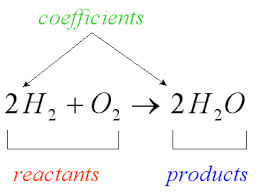Balancing Equation: We balance a chemical equation so that no. of atoms of each element involved in the reaction remains the same at the reactant and product side.

$Fe+{H}_{2}O\to FeO+{H}_{2}$
$3Fe+4{H}_{2}O⟶F{e}_{3}{O}_{4}+4{H}_{2}$

Types of Reaction

I. Combination Reaction:– The reaction in which two or more substances combine to form a new single substance.
e.g.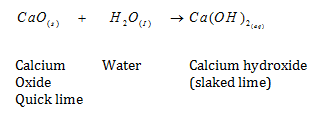• ${C}_{a}\left(OH{\right)}_{2}\phantom{\rule{thickmathspace}{0ex}}$ slaked lime is used for white washing walls. It reacts with $C{O}_{2}$ to form Calcium Carbonate and gives a shiny finish to the walls

$Ca\left(OH{\right)}_{{2}_{\left(aq\right)}}+C{{O}_{2}}_{\left(g\right)}\to \phantom{\rule{0ex}{0ex}}CaC{O}_{{3}_{\left(s\right)}}+{H}_{2}O\left(l\right)$

Calcium Hydroxide Calcium Carbonate

(ii) Burning of Coal

${C}_{\left(2\right)}+{O}_{{2}_{\left(g\right)}}\phantom{\rule{thickmathspace}{0ex}}\to C{O}_{{2}_{\left(g\right)}}\phantom{\rule{thickmathspace}{0ex}}+$heat+ light

(iii) Formation of water

$2{H}_{{2}_{\left(g\right)}}\phantom{\rule{thickmathspace}{0ex}}+\phantom{\rule{thickmathspace}{0ex}}{O}_{2\left(g\right)}\phantom{\rule{thickmathspace}{0ex}}\to \phantom{\rule{thickmathspace}{0ex}}2{H}_{2}O\left(l\right)$

Exothermic Reactions : Reaction in which heat is released along with the formation of products.

eg.. $\phantom{\rule{thickmathspace}{0ex}}\phantom{\rule{thickmathspace}{0ex}}C{H}_{{4}_{\left(g\right)}}+\phantom{\rule{thickmathspace}{0ex}}2{O}_{{2}_{\left(g\right)}}\phantom{\rule{thickmathspace}{0ex}}\phantom{\rule{thickmathspace}{0ex}}\to \phantom{\rule{thickmathspace}{0ex}}C{O}_{{2}_{\left(g\right)}}+\phantom{\rule{thickmathspace}{0ex}}2{H}_{2}{O}_{\left(g\right)}$

Endothermic Reaction :The reactions which require energy in form of heat, light or electricity are called endothermic reaction

eg..

II. Decomposition Reactions :The reaction in which a single substance decomposes to give two or more substances. De composition reactions can be of three types:

DECOMPOSITION REACTIONS :-

1. Thermal Decompostition :-When a decomposition reaction is carried out by heating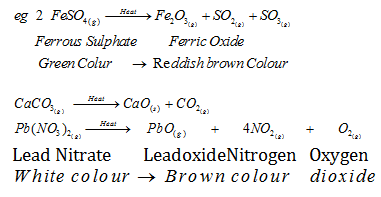1. Electrolytic Decomposition :- When a decomposition reaction is carried out by electric current,
2. .${H}_{{2}_{\left(l\right)}}\phantom{\rule{thickmathspace}{0ex}}\phantom{\rule{thickmathspace}{0ex}}\underset{current}{\overset{electric}{\to }}2{H}_{{2}_{\left(g\right)}}+\phantom{\rule{thickmathspace}{0ex}}{O}_{{2}_{\left(g\right)}}$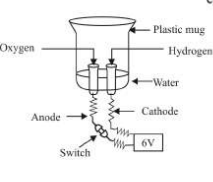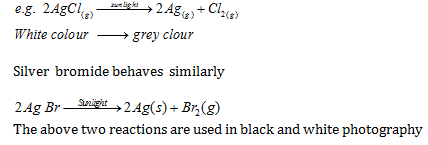• Silver bromide behaves similarly

$2Ag\phantom{\rule{thickmathspace}{0ex}}Br\phantom{\rule{thickmathspace}{0ex}}\stackrel{Sunlight}{\to }\phantom{\rule{thickmathspace}{0ex}}2Ag\left(s\right)\phantom{\rule{thickmathspace}{0ex}}+\phantom{\rule{thickmathspace}{0ex}}B{r}_{2}\left(g\right)$

III. Displacement Reaction:The chemical reaction in which an element displaces another element from its solution

$F{e}_{\left(s\right)}+CuS{O}_{{}_{4\left(aq\right)}}\to FeS{O}_{4}+C{u}_{\left(s\right)}$

Copper (aq)

Sulphate Iron Sulphate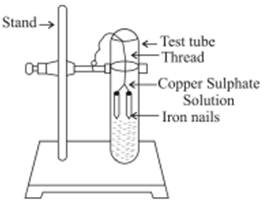Fe being more reactive than Cu displaces it from ${C}_{u}S{O}_{4}$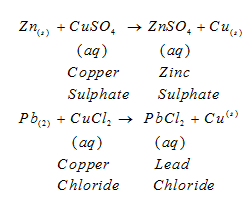• Zinc and lead are more reactive elements than copper. They displace copper from its compounds.

IV. Double Displacement Reaction :The reaction in which two different atoms or group of atoms are mutually exchanged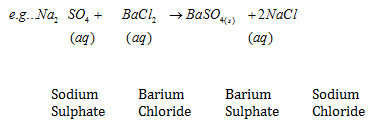A white substance is formed due to above reaction. The insoluble substance i.e., $BaS{O}_{4}$is called precipitate.

Precipitation Reaction – Any reaction that produces a precipitate is called a precipitation reaction.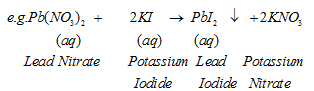the downward facing arrown represents the formation of precipitate.

V. Oxidation :Oxidation is the gain of oxygen or loss of hydrogen

$e.g.\phantom{\rule{thickmathspace}{0ex}}\phantom{\rule{thickmathspace}{0ex}}\phantom{\rule{thickmathspace}{0ex}}2Cu\phantom{\rule{thickmathspace}{0ex}}+\phantom{\rule{thickmathspace}{0ex}}{O}_{2}\phantom{\rule{thickmathspace}{0ex}}\stackrel{Heat}{\to }\phantom{\rule{thickmathspace}{0ex}}\phantom{\rule{thickmathspace}{0ex}}2CuO$

When copper is heated a black colour appears. If this CuO is reactedwith hydrogen gas then again Cu becomes brown as reverse reaction takes place

${C}_{u}O\phantom{\rule{thickmathspace}{0ex}}+\phantom{\rule{thickmathspace}{0ex}}{H}_{2}\phantom{\rule{thickmathspace}{0ex}}\stackrel{Heat}{\to }\phantom{\rule{thickmathspace}{0ex}}Cu\phantom{\rule{thickmathspace}{0ex}}+{H}_{2}O$

VI. Reduction : Reduction is the loss of oxygen or gain of hydrogen.

• Redox Reaction : The reaction in which one reactant gets oxidised while other gets reduced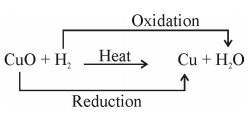eg.. $ZnO+\phantom{\rule{thickmathspace}{0ex}}C\phantom{\rule{thickmathspace}{0ex}}\to \phantom{\rule{thickmathspace}{0ex}}\phantom{\rule{thickmathspace}{0ex}}Zn\phantom{\rule{thickmathspace}{0ex}}+\phantom{\rule{thickmathspace}{0ex}}CO$

$Mn{O}_{2}+4HCl\to MnC{l}_{2}+2{H}_{2}O+C{l}_{2}$

• Corrosion :When a metal is attacked by substances around it such as moisture, acids etc.

(i) Rusting of iron. i.e Reddish brown coating on iron of $F{e}_{2}{O}_{3}$isformed.

(ii) Black coating on Silver.

Rusting of iron can be prevented by painting, oiling the surface or by galvanisation.

• Rancidity :When fats and oils are oxidised they become rancidand their smell and taste change.
• Antioxidants are added to foods containing fats and oil.

e.g.${N}_{2}$is added to packet of chips to prevent oxidation of fats and oils.

What you have learnt

1. A complete chemical equation represents the reactants, products and their physical states symbolically.
2. A chemical equation is balanced so that the numbers of atoms of each type involved in a chemical reaction are the same on the reactant and product sides of the equation. Equations must always be balanced.
3. In a combination reaction two or more substances combine to form a new single substance.
4. Decomposition reactions are opposite to combination reactions. In a decomposition reaction, a single substance decomposes to give two or more substances.
5. Reactions in which heat is given out along with the products are called exothermic reactions.
6. Reactions in which energy is absorbed are known as endothermic reactions.
7. When an element displaces another element from its compound, a displacement reaction occurs.
8. Two different atoms or groups of atoms (ions) are exchanged in double displacement reactions.
9. Precipitation reactions produce insoluble salts.
10. Reactions also involve the gain or loss of oxygen or hydrogen by substances.Oxidation is the gain of oxygen or loss of hydrogen. Reduction is the loss of oxygenor gain of hydrogen.